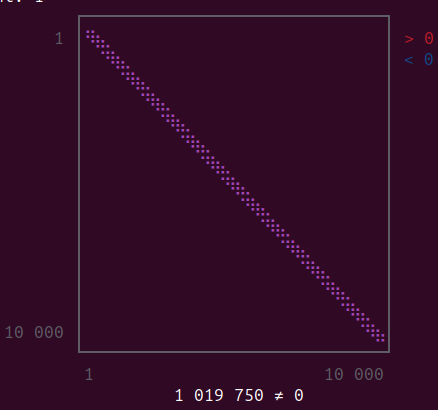# Is it possible to parallelize matrix division

I have a big (~10000x10000) but sparse matrix A and a vector B. I want to solve the system using backslash

C = A\B ,

and it works, but it is taking too long. Is there a way to parallelize this and utilize multiple cores on the computer?

It’s possible that the default sparse solver may be parallel already (I’m not sure), or at least to the extent it’s possible/useful for the problem and algorithm.

But you might look into other solvers based on different algorithms. Take a look at some of the packages suggested in this thread. Whether or not they’re parallel, they may offer a speed benefit depending on your problem.

1 Like

Thanks a lot. UMFPACKFactorization() and KLUFactorization() improve the performance, but I do not see them parallelizing the code. I have checked that BLAS.get_num_threads() returns 4, but only one CPU is working on the problem. I have access to a computer with 64 cores, and it would be great if I could utilize it. I am planning to run a calculation with a much bigger size of matrices.

The following code works well and gives a fantastic multithreading speedup. For some reason this code only works for julia version 1.8.2 and not for julia version 1.9.2. I get an error saying “reordering problem”.
I think there is a bug in julia 1.9.2. Where should I report this?

using Pardiso
using SparseArrays
using Random
using Printf
using Test
# -----------------
verbose = false
n = 10000
A = sprand(n, n, 0.01)
B = rand(n)

# Initialize the PARDISO internal data structures.
# ps = PardisoSolver()
ps = MKLPardisoSolver()
set_nprocs!(ps, 4)
set_iparm!(ps, 12, 1)
println(get_iparm(ps, 12))
if verbose
set_msglvl!(ps, Pardiso.MESSAGE_LEVEL_ON)
end

# If we want, we could just solve the system right now.
# Pardiso.jl will automatically detect the correct matrix type,
# solve the system and free the data
X1 = solve(ps, A, B)

# We also show how to do this in incremental steps.
#println(X1)

2 Likes

Apologies for the drive-by, but I’m not at my computer right now. You might be interested in this video by @ChrisRackauckas .

That should just be MKLPardisoFactorize() / MKLPardisoIterate() in LinearSolve.jl . Try those and see if it works well.

UMFPACK does parallelize, but OpenBLAS doesn’t use that many threads that well. If you use using MKL then UMFPACK may use threads better (and don’t forget to BLAS.set_num_threads(64))

1 Like

1 Like

BTW @Shashank if you can provide the setup for the matrix then we could add it to the SciMLBenchmarks to better track performance of this kind of case. Right now the best I could point to are the two benchmarks we have there:

1 Like

Thanks Chris. I am trying to solve a linear integro-pde

Dr = Differential(r)
Dcth = Differential(cth)
Icth = Integral(cth in DomainSets.OpenInterval(-1,1))

# 2D PDE
eq = cth * Dr(u(r,cth)) + ((1 - cth * cth) / r) * Dcth(u(r,cth)) ~ cemit(r) - d(r) * u(r,cth) + cd(r) * Icth(u(r,cth))


where cemit(r), d(r) and cd(r) are functions that are inputs.
This is just a steady-state solution for a transport equation in spherical geometry. Since this is a linear equation, it is easy to calculate the Jacobian. If we take a grid over r and \cos\theta that has 100 bins each, the jacobian is 10,000 by 10,000. Due to the integral in the equation, the Jacobian is not tridiagonal but broader. The sparsity pattern is shown below,Instead of O(30000) one would expect in a normal pde, I have about a million non-zero entries in the Jacobian. I am working on a project that involves adding nonlinear corrections to this equation, but right now, I am trying to figure out an optimal way of solving the linear part so that I can know which solvers work well in which situation. For now, it seems Pardiso.jl is the best. But I will make plots for all the methods I have tried and post them here so that we have a benchmark for integro-differential equations using single and multiple threads. I was also planning to do the same using a GPU node on the cluster to see whether that gives an advantage in this case.

Is that not a banded matrix? If it’s a banded matrix you should specify it with BandedMatrices.jl and fully specialize on that.

I did not know this was available. I will try that too. Thanks a lot.